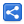# Java 8 - Arrays enhancements

[Last Updated: Sep 11, 2016]

In Java 8, `java.util.Arrays` class has been enhanced with various static factory methods like parallel operation, accumulative operations, element generator operations, creating java.util.stream.Stream or other primitive stream equivalents and converting a given array into java.util.Spliterator.

## Parallel sort operations

#### void parallelSort (Ar[] array, ....)

These overloaded methods perform sorting of the provided arrays using Fork and Join framework, processing sorting algorithm in parallel.

Ar can be any primitive or any object type.

The overloaded methods can accept fromIndex and toIndex to sort only in provided range.

If Ar is other than primitive types, then we can provide a Comparator object as parameter.

There's another overloaded method which can accept Ar as being Comparable.

```   int [] intArray = {3,1,24,5,4,61,59,7,15,3,12,22};
Arrays.parallelSort(intArray);
System.out.println(Arrays.toString(intArray));```

In above example, we used a very small array size for brevity. Generally speaking, Parallel operations are beneficial for large array size otherwise we should use one of their counterpart Arrays#sort methods.

## Parallel accumulative operations

#### void parallelPrefix(Ar[] array, ...., SomeBinaryOperator op)

These overloaded methods cumulate, in parallel, each element of the given array in place, using the supplied SomeBinaryOperator

Ar can be long, int, double or any other object type.

In case if Ar is long, int or double, we use corresponding LongBinaryOperator, IntBinaryOperator or DoubleBinaryOperator respectively as the last non-optional parameter.

In case if Ar is object type, we use BinaryOperator

Here too we can optionally specify fromIndex and toIndex as the operation range.

```   int [] intArray = {1,2,3,4,5};
Arrays.parallelPrefix(intArray, (left, right) -> left + right);
System.out.println(Arrays.toString(intArray));
```

## Sequential and Parallel Array generator operations

#### void parallelSetAll(Ar[] array, SomeFunction func)

Set all elements of the specified array, using the provided generator function to compute each element.

Ar can be any int, long or double or object type

SomeFunction can be IntUnaryOperator, IntToLongFunction or IntToDoubleFunction or for object type it can be IntFunction

```   int [] intArray = new int;
Arrays.setAll(intArray, index -> index*2);
System.out.println(Arrays.toString(intArray));```

```   BigDecimal[] bds = new BigDecimal;
Arrays.parallelSetAll(bds, index -> new BigDecimal(index));
System.out.println(Arrays.toString(bds));```

## Creating streams

#### DoubleStream stream(double[] array)

Each above method has a overloaded startInclusive and endExclusive version as well.

```  int[] ints = new int;
Arrays.fill(ints, 10);
int sum = Arrays.stream(ints)
.map(i -> (int) (Math.random() * i))
.sum();
System.out.println(sum);```

## Creating Spliterator

#### Spliterator.OfDouble spliterator(double[] array)

Returns a Spliterator covering all or provided range of the specified array.

Each above method has a overloaded startInclusive and endExclusive version as well.

```  int[] ints = new int;
Arrays.fill(ints, 10);
Spliterator.OfInt spliterator = Arrays.spliterator(ints);
spliterator.forEachRemaining((IntConsumer) System.out::println);```

 Share